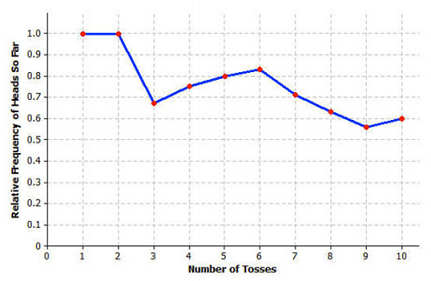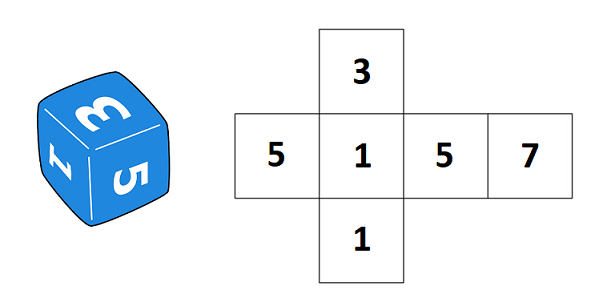# Probability

## Objective

Use probability to predict long-run frequencies.

## Common Core Standards

### Core Standards

?

• 7.SP.C.6 — Approximate the probability of a chance event by collecting data on the chance process that produces it and observing its long-run relative frequency, and predict the approximate relative frequency given the probability. For example, when rolling a number cube 600 times, predict that a 3 or 6 would be rolled roughly 200 times, but probably not exactly 200 times.

?

• 7.RP.A.3

## Criteria for Success

?

1. Use experimental and theoretical probabilities to make predictions of long-run frequencies or larger populations.
2. Understand that long-run frequencies tend to approximate theoretical probability.
3. Understand that predictions are reasonable estimates and not exact measures.

## Tips for Teachers

?

Students draw on their ratio and proportion reasoning skills to make predictions of long-run frequencies. This is a good opportunity to review or re-engage students in ratio reasoning skills from Units 1 and 5.

#### Fishtank Plus

• Problem Set
• Student Handout Editor
• Vocabulary Package

## Anchor Problems

?

### Problem 1

Alexander flips a coin 3 times. It lands on heads 0 times.

a.  Do you think this is a fair coin? Explain why or why not.

Alexander flips the same coin 100 times. It lands on heads 47 times.

b.  Do you think this is a fair coin? Explain why or why not.

c.   If Alexander were to flip the coin 500 times, about how many times would you expect the coin to land on heads?

Taylah flips a coin and creates the graph below to represent her results.d.   Explain what the graph tells you about Taylah’s experiment.

e.   Does it appear that Taylah used a fair coin? Explain your answer.

#### References

EngageNY Mathematics Grade 7 Mathematics > Module 5 > Topic B > Lesson 8Image from Example 1e

Grade 7 Mathematics > Module 5 > Topic B > Lesson 8 of the New York State Common Core Mathematics Curriculum from EngageNY and Great Minds. © 2015 Great Minds. Licensed by EngageNY of the New York State Education Department under the CC BY-NC-SA 3.0 US license. Accessed Dec. 2, 2016, 5:15 p.m..

### Problem 2

On a game show, contestants spin a wheel to determine which game, of four options, they will play to win a prize. The games of the last several contestants are shown below.

 Game Frequency Guess the Price 16 Match the Brand 7 Throw the Darts 5 Race the Clock 12

About how many of the next 200 contestants do you expect will play Race the Clock? Explain your reasoning.

### Problem 3

A six-sided number cube includes the numbers 1, 3, 5, and 7, as shown below.Han predicts that out of 150 rolls of the number cube, the number 1 will appear exactly 50 times.

Do you agree with Han? Explain your reasoning.

## Problem Set

?The following resources include problems and activities aligned to the objective of the lesson that can be used to create your own problem set.